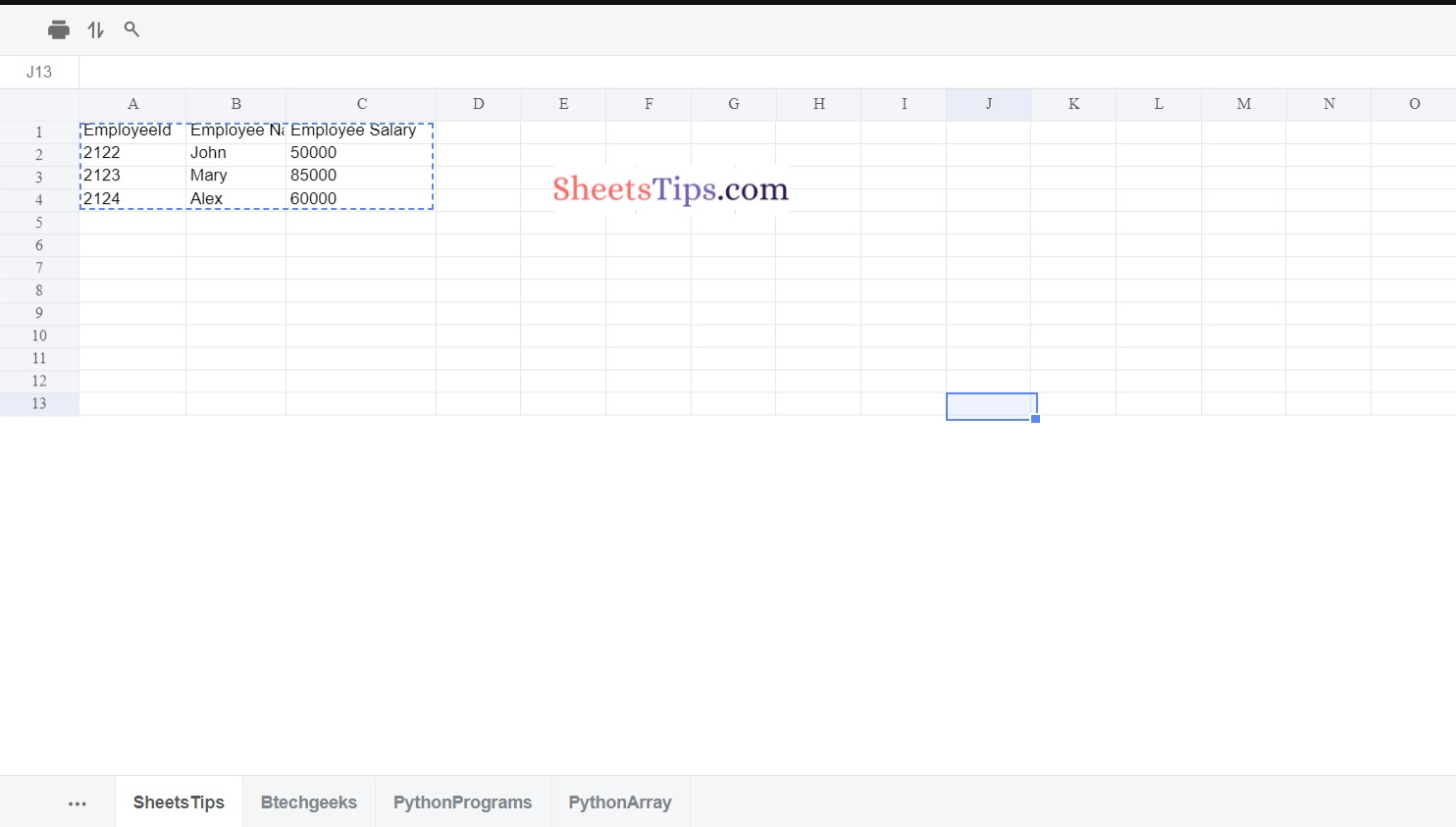# How to Create Sheets in Excel File in Java using Apache POI?

Because an excel worksheet uses cells to store data, reading and writing an excel file with Java is a bit challenging. Java does not include an API for reading or writing Microsoft Excel or Word documents. We must rely on the Apache POI third-party library.

In this post, let us look at how to create an excel file in Java and how to write or insert data into it with the Apache POI Java library.

Apache POI library in Java:

Apache POI is a free and open-source Java library for creating and manipulating Microsoft Office file formats. The following file formats should be able to be created, modified, and displayed/read using POI. For example, because java does not include built-in support for interacting with Excel files, we must search for open-source APIs to complete the job.

Apache POI provides a Java API for handling multiple file formats based on Microsoft’s Office Open XML (OOXML) and OLE2 standards. The Apache License governs the distribution of Apache POI releases (V2.0).

The Apache POI (Poor Obfuscation Implementation) Java API is used to read and write Microsoft documents. It includes interfaces and classes. For reading or writing excel files, the Apache POI library provides two implementations:

Implementation of HSSF (Horrible SpreadSheet Format): It implies an API that works with Excel 2003 or earlier versions.
Implementation of XSSF (XML SpreadSheet Format): It denotes an API that works with Excel 2007 or later versions.

## Creating Sheets in Excel File in Java using Apache POI

To create an excel file using apache poi we should first create a maven project. You can either use MyEclipse or STS(SpringToolSuite) for this. Here I am using STS

STS:

Spring Tool Suite (STS) is a Java IDE designed specifically for the development of Spring-based enterprise applications. It is simpler, quicker, and more convenient. Most notably, it is built on the Eclipse IDE. STS is a free, open-source software platform powered by VMware. Spring Tools 4 represents the next generation of Spring tooling for your preferred development environment. It was largely redesigned from the bottom up to give world-class support for developing Spring-based enterprise apps, regardless of whether you choose Eclipse, Visual Studio Code, or Theia IDE.

Note:

`If you are using MyEclipse then create a maven project.`

STS directly imports all the classes from maven if we just add dependencies.

Step#1: Open STS(SpringToolSuite) and create a spring stater project say SampleExcelFile in a package com.sheetstips as shown below.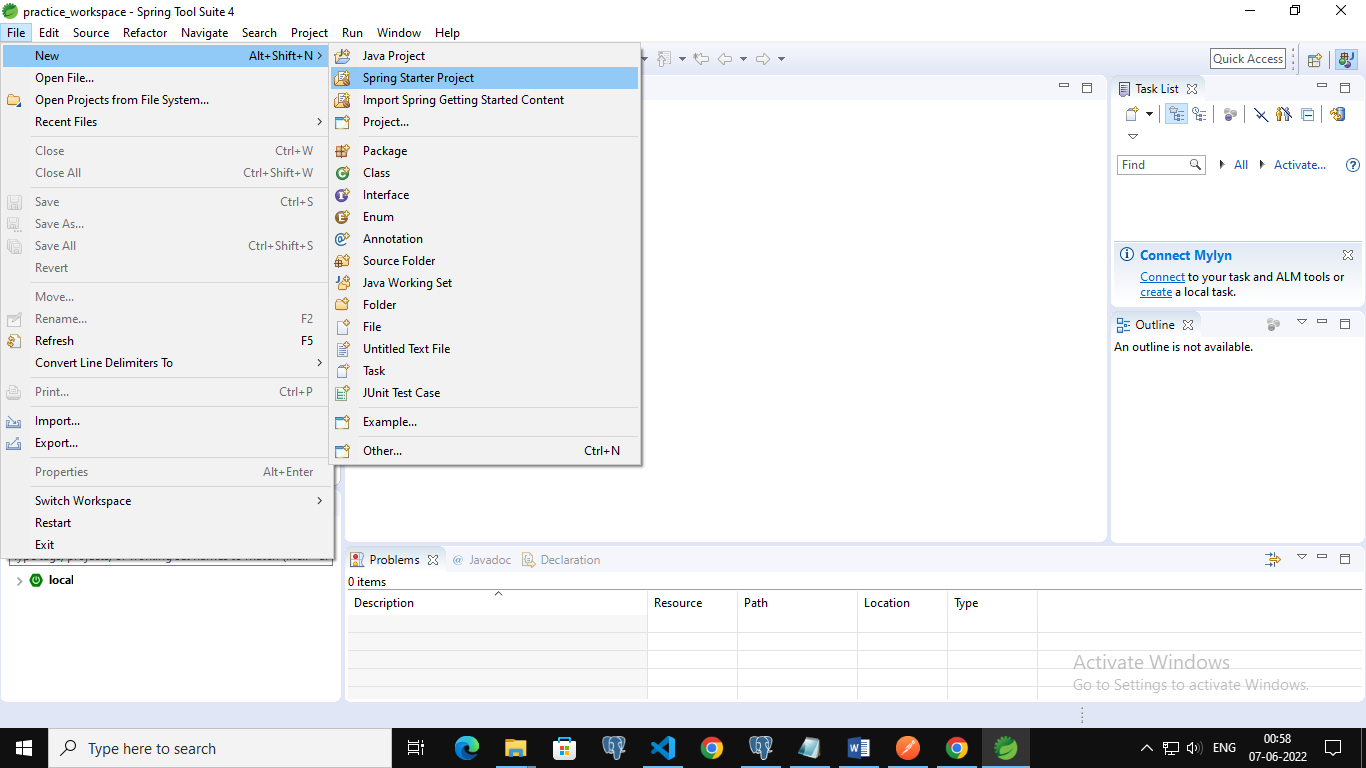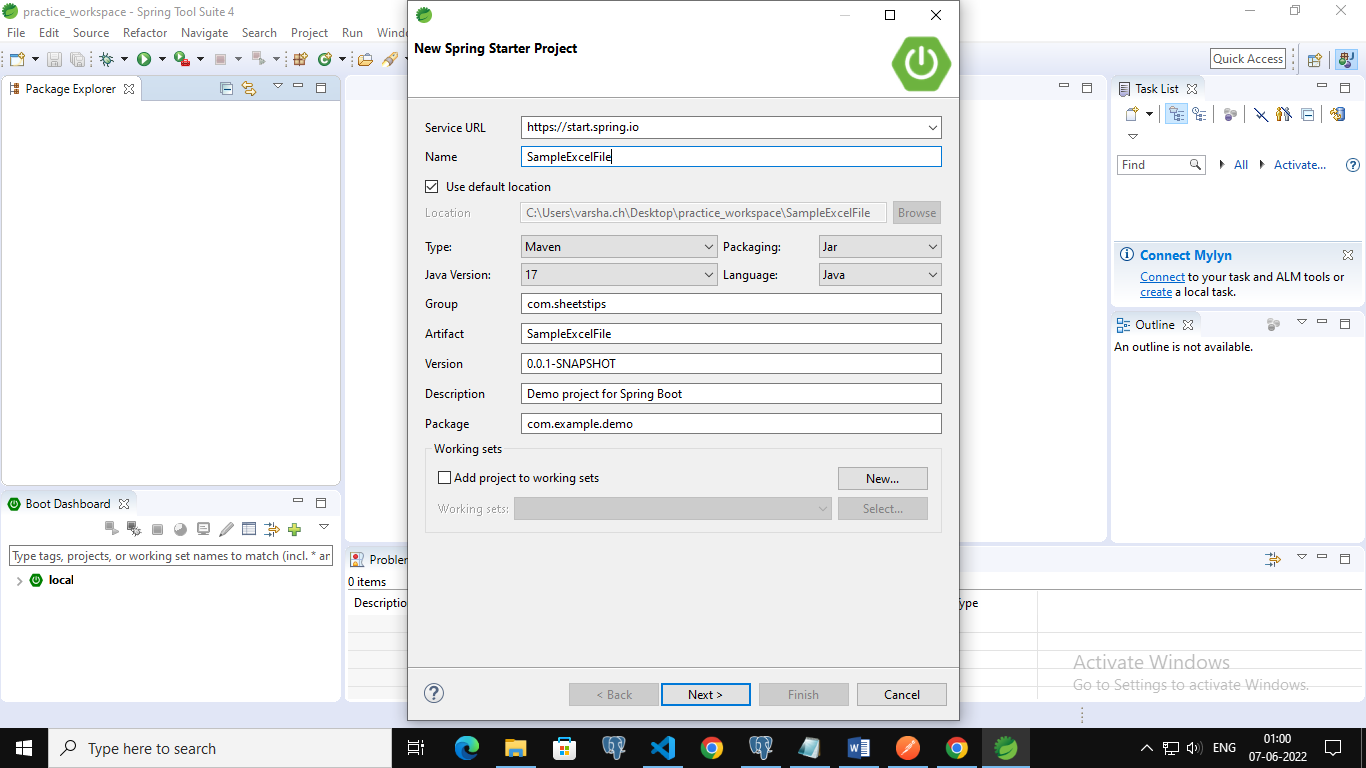Step#2: Add the spring web dependency and click on Finish. Now the project is created successfully.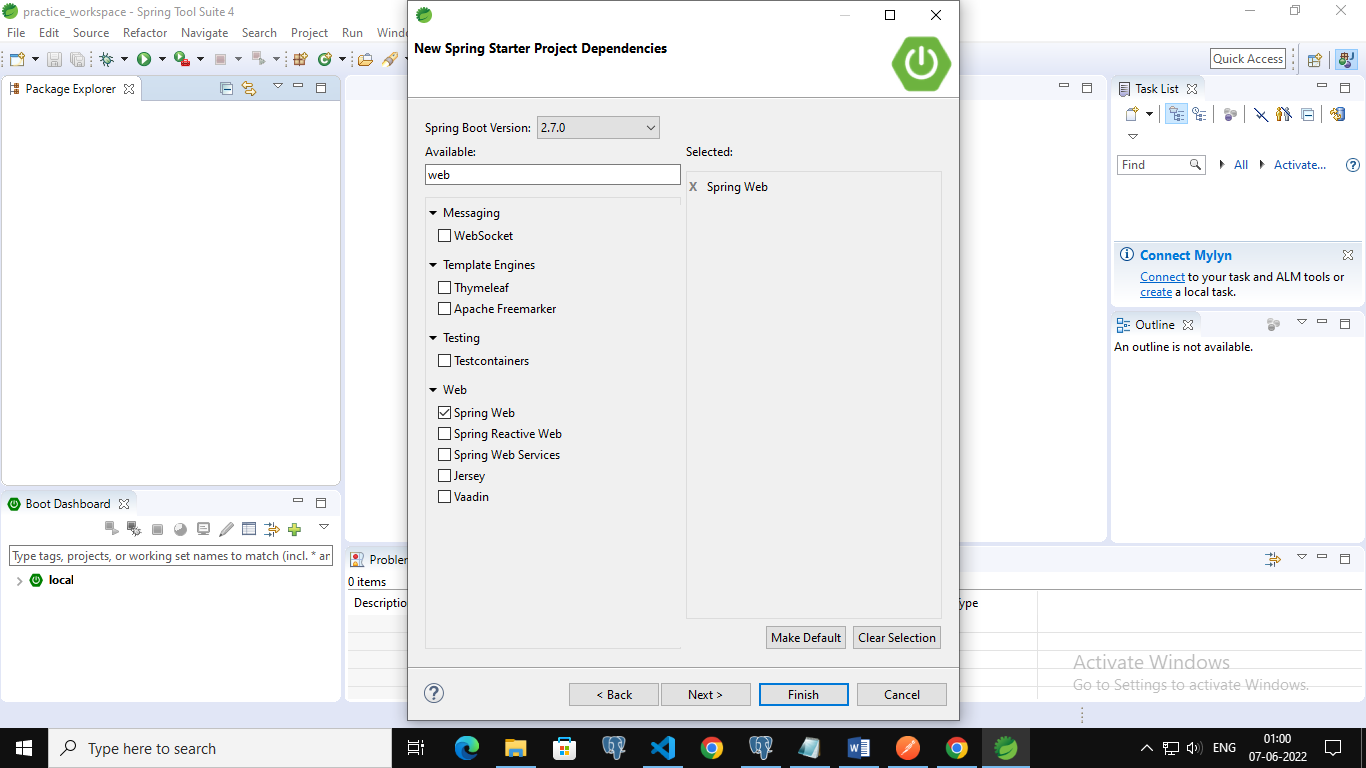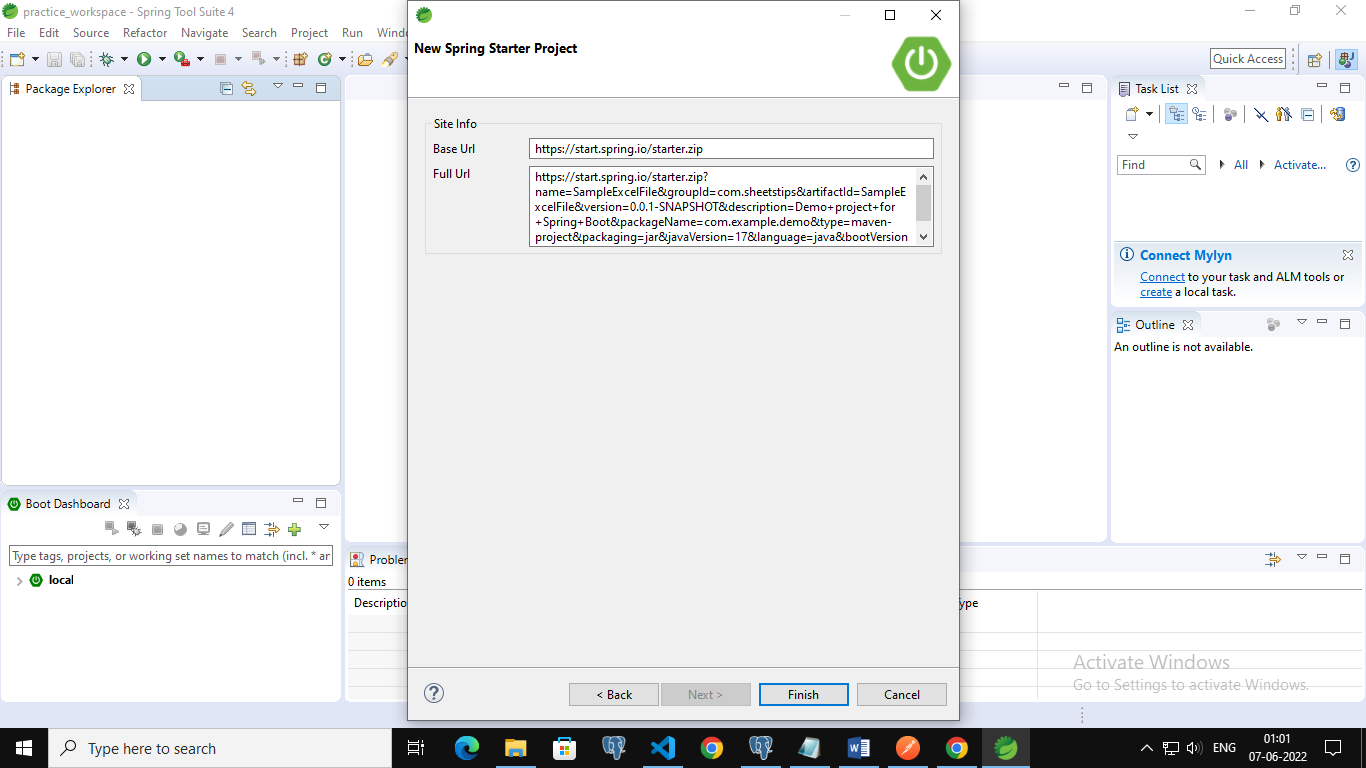Step#3: Open the pom.xml file and add the following apache poi dependency.

https://mvnrepository.com/artifact/org.apache.poi/poi

```<dependency>
<groupId>org.apache.poi</groupId>
<artifactId>poi</artifactId>
<version>3.12</version>
</dependency>
<dependency>
<groupId>org.apache.poi</groupId>
<artifactId>poi-ooxml</artifactId>
<version>3.12</version>
</dependency>```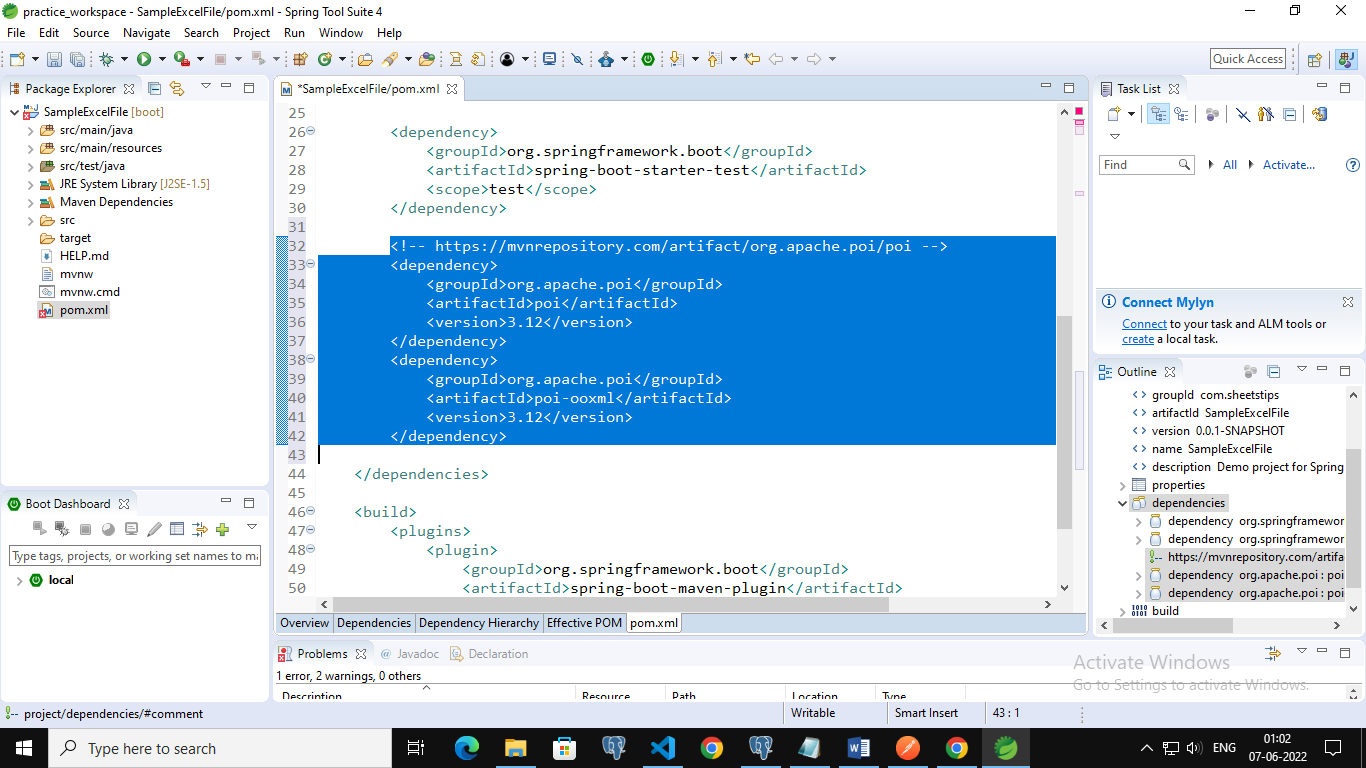Step#4: Create a new class say DemoExcelFile in the src/main/java folder with the same package com.sheetstips. But here I have changed the package name to com.example.demo.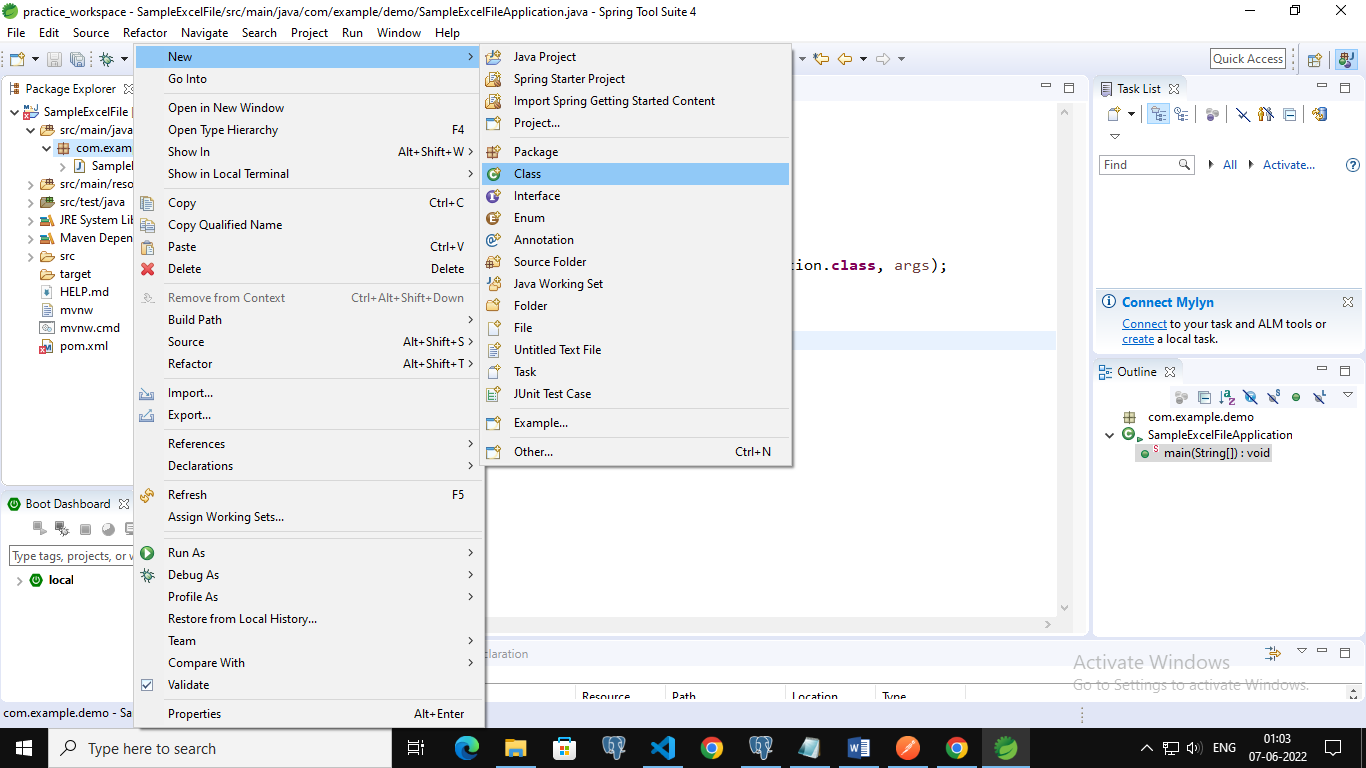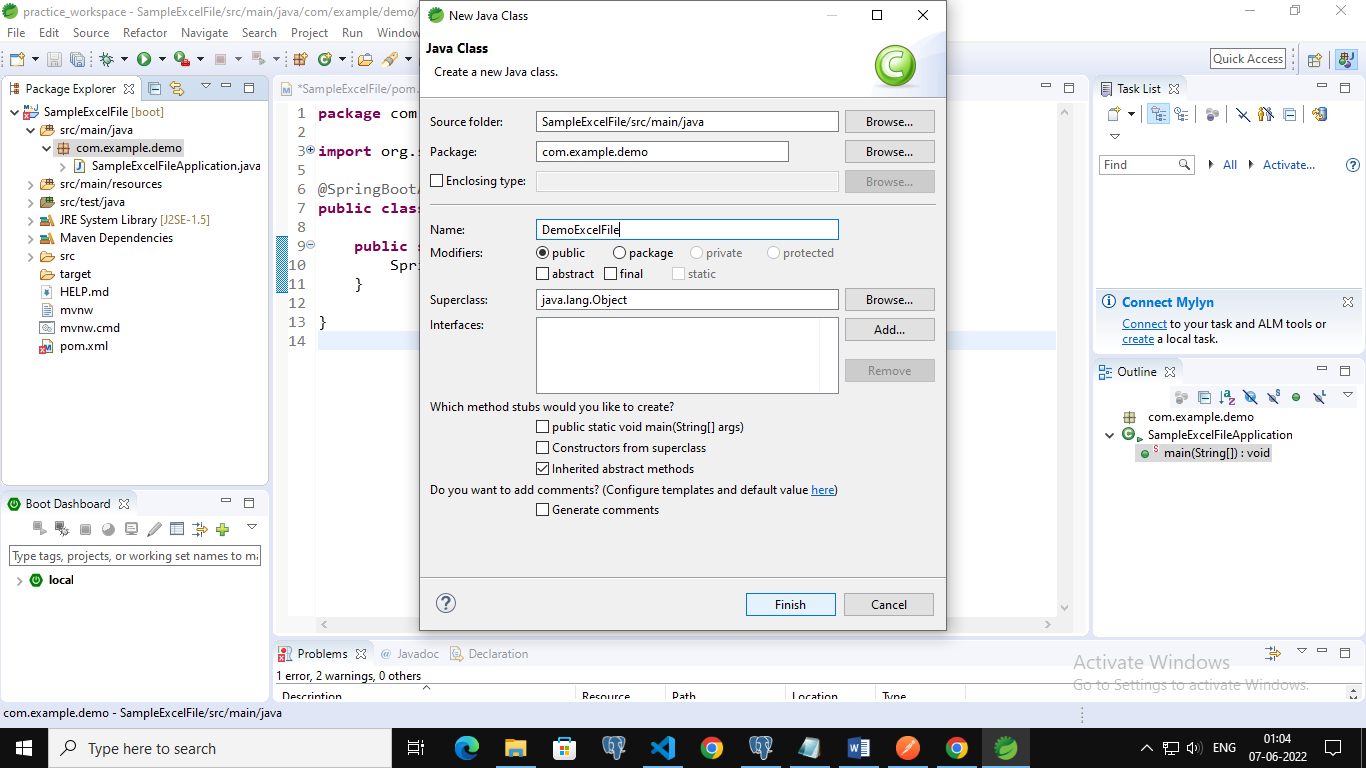Step#5: Write the below code in the above DemoExcelFile class

Approach:

• Import all the required classes using the import keyword
• Press ctri+shft+o to import directly
• Create a class say DemoExcelFile
• Inside the Main driver method, create a new HSSFWorkbook object
• Create an object for the FileOutputStream by passing the output excel file name as an argument to it
• The output stream accepts the output bytes and sends them to sink
• Create sheets of an excel file using the createSheet() function by applying it on the above workbook object
• Print some random text for acknowledgment on the console which represents the successful creation of an excel file.
• Print the total number of sheets present in the Excel File on the console using the getNumberOfSheets() function.
• Write the above output Excel File into the workbook object using the write() function.
• The Exit of the Program.

Below is the implementation:

```package com.example.demo;

//Import all the required classes using the import keyword
// Press ctri+shft+o to import directly
import java.io.FileNotFoundException;
import java.io.FileOutputStream;
import java.io.IOException;
import java.io.OutputStream;

import org.apache.poi.hssf.usermodel.HSSFWorkbook;
import org.apache.poi.ss.usermodel.Sheet;
import org.apache.poi.ss.usermodel.Workbook;

//Create a class say DemoExcelFile
public class DemoExcelFile {

// Main driver method
public static void main(String[] args)
throws FileNotFoundException, IOException
{
// Create a new HSSFWorkbook object
Workbook workbookObj = new HSSFWorkbook();

// Create an object for the FileOutputStream by passing the output excel file
// name as an argument to it
// The output stream accepts the output bytes and sends them to sink
OutputStream outputExcelFile
= new FileOutputStream("sampleExcelFile.xlsx");

// Create sheets of an excel file using the createSheet() function
// by applying it on the above workbook object
Sheet sheet1 = workbookObj.createSheet("SheetsTips");
Sheet sheet2 = workbookObj.createSheet("Btechgeeks");
Sheet sheet3 = workbookObj.createSheet("PythonPrograms");
Sheet sheet4 = workbookObj.createSheet("PythonArray");

//Print some random text for acknowledgment which represents
// the successful creation of an excel file
System.out.println(
"Hey! Excel Sheet is created successfully!!!!!");

// Print the total number of sheets present in the Excel File
// on console using the getNumberOfSheets() function
int TotalnumOf_sheets = workbookObj.getNumberOfSheets();
System.out.println("No of Sheets present in an Excel File = "
+ TotalnumOf_sheets);

// Write the above output Excel File into the workbook object
// using the write() function
workbookObj.write(outputExcelFile);
}
}```

Step#5: Now run the code by right-clicking on the project and pressing run as springboot App

Output:

```Hey! Excel Sheet is created successfully!!!!!
No of Sheets present in an Excel File = 4```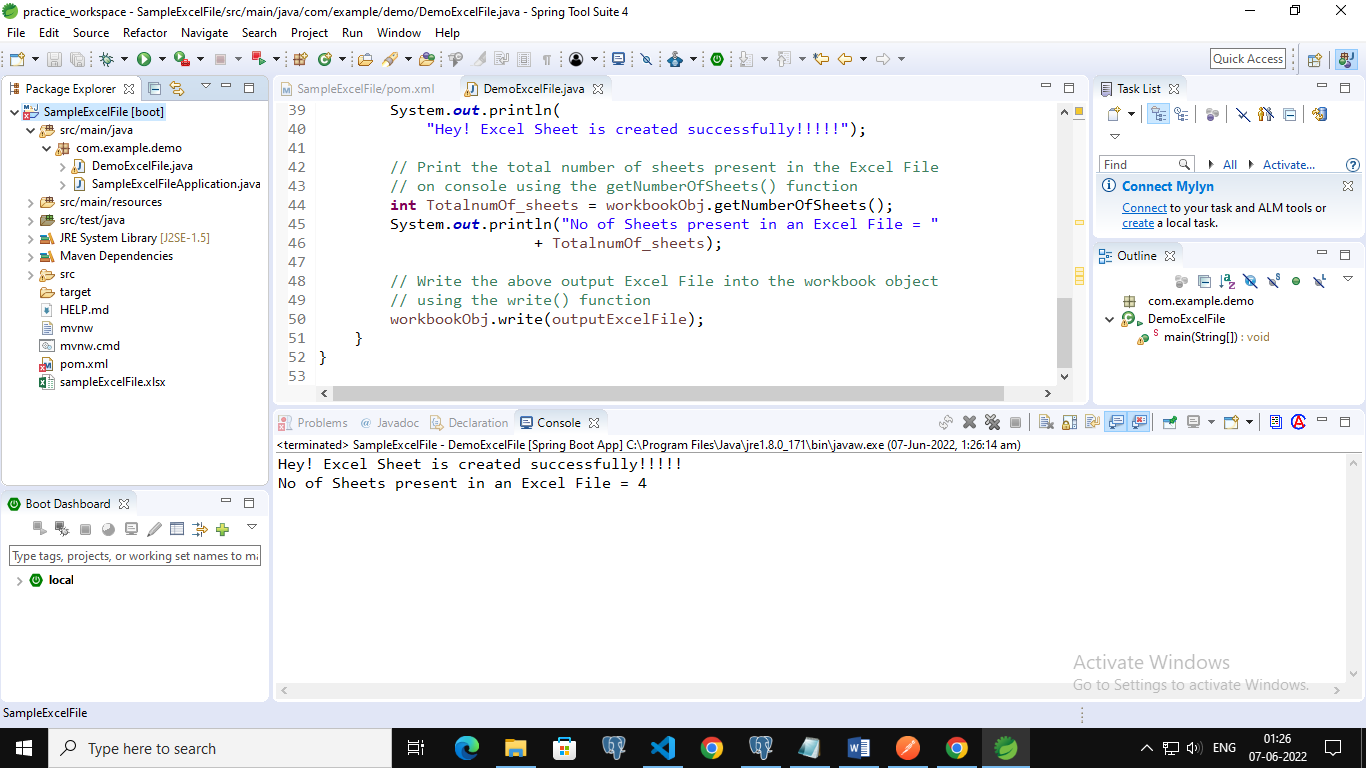Step#6: Now when we refresh the project we can observe that a new excel file with the name SampleExcelFile is created which is the output file that we want.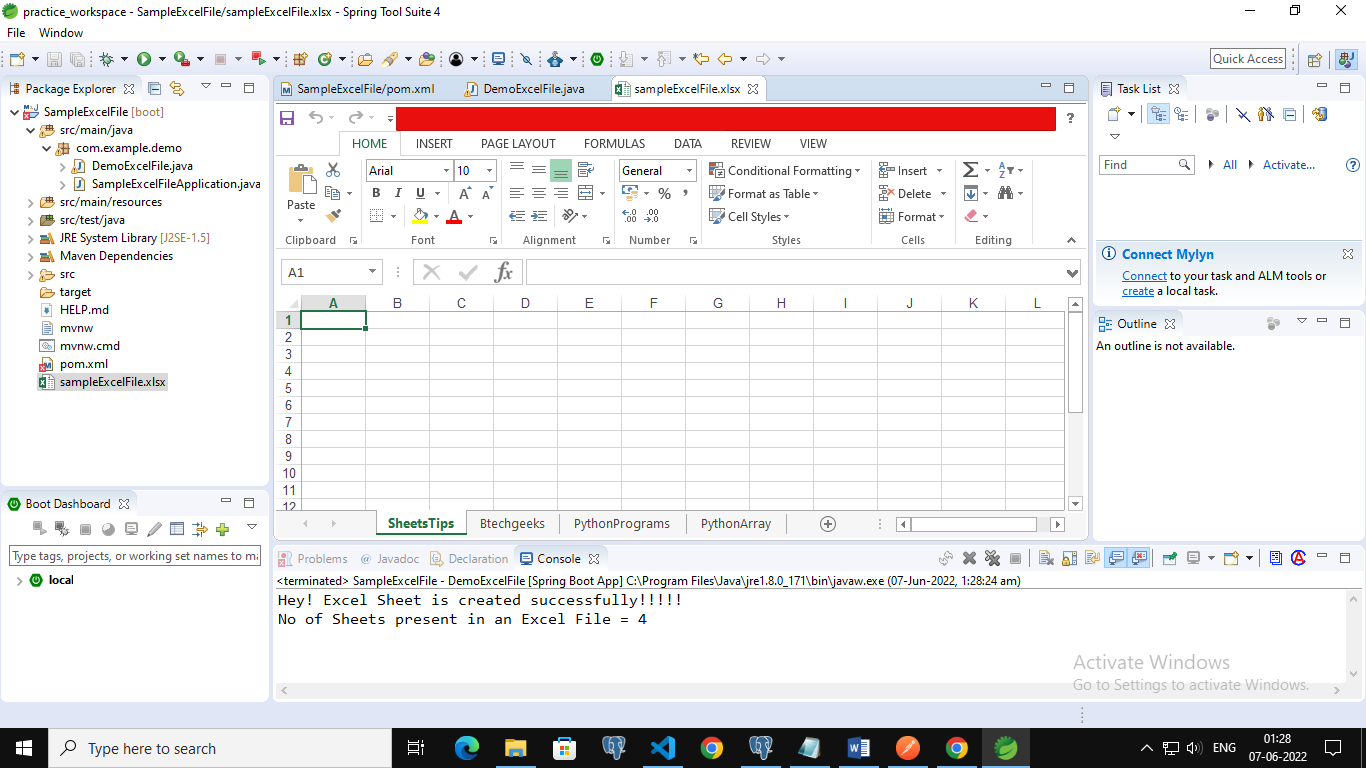Explanation:

``` A new excel file is created containing 4 sheets say SheetsTips, Btechgeeks,
PythonPrograms and PythonArray```

### Inserting Data into the Sheets of an Excel File

Approach:

• Import all the required classes using the import keyword
• Press ctri+shft+o to import directly
• Create a class say DemoExcelFile
• Inside the Main driver method, create a new HSSFWorkbook object
• Create an object for the FileOutputStream by passing the output excel file name as an argument to it
• The output stream accepts the output bytes and sends them to sink.
• Create sheets of an excel file using the createSheet() function by applying it in the above workbook object.
• Create sheets of an excel file using the createSheet() function by applying it in the above workbook object.
• Insert data into the sheet1(SheetsTips) as follows.
• Create 0th row using the createRow() function, and HSSFRow object
• Insert data into the 0th row by creating cell values using the createCell() function and set the values to the corresponding cell using the setCellValue() function
• Create 1st row using the createRow() function, and HSSFRow object
• Insert data into the 1st row by creating cell values using the createCell() function and set the values to the corresponding cell using the setCellValue() function
• Create 2nd cell and insert data into that cell
• Similarly, create 3rd cell and insert data into that cell inserting data in the second row
• In the same way as above create second sheet into the same workboot object using the createSheet() function and insert data into the worksheet as shown above.
• Insert data into sheet2(Btechgeeks) as follows.
• Create 0th row in the sheet2 using the createRow() function, and HSSFRow object
• Insert data into the 0th row by creating cell values using the createCell() function and set the values to the corresponding cell using the setCellValue() function.
• Create 1st row in the sheet2 using the createRow() function.
• Insert data into the 1st row by giving the cell values.
• Create 2nd row in the sheet2 using the createRow() function
• Insert data into the 2nd row by giving the cell values
• Similarly, create 3rd cell and insert data into the cells
• Print some random text for acknowledgment that represents successful creation of excel file
• Print the total number of sheets present in the Excel File on console using the getNumberOfSheets() function
• Write the above output Excel File into the workbook object using the write() function.
• The Exit of the Program.

Below is the implementation:

```package com.example.demo;

//Import all the required classes using the import keyword
// Press ctri+shft+o to import directly
import java.io.FileNotFoundException;
import java.io.FileOutputStream;
import java.io.IOException;
import java.io.OutputStream;

import org.apache.poi.hssf.usermodel.HSSFRow;
import org.apache.poi.hssf.usermodel.HSSFWorkbook;
import org.apache.poi.ss.usermodel.Row;
import org.apache.poi.ss.usermodel.Sheet;
import org.apache.poi.ss.usermodel.Workbook;

//Create a class say DemoExcelFile
public class DemoExcelFile {

// Main driver method
public static void main(String[] args) throws FileNotFoundException, IOException {
// Create a new HSSFWorkbook object
Workbook workbookObj = new HSSFWorkbook();

// Create an object for the FileOutputStream by passing the output excel file
// name as an argument to it
// The output stream accepts the output bytes and sends them to sink
OutputStream outputExcelFile = new FileOutputStream("sampleExcelFile.xlsx");

// Create sheets of an excel file using the createSheet() function
// by applying it in the above workbook object
Sheet sheet_1 = workbookObj.createSheet("SheetsTips");

// Insert data into the sheet1(SheetsTips) as follows.
// Create 0th row using the createRow() function, and HSSFRow object
HSSFRow row_0 = (HSSFRow) sheet_1.createRow((short) 0);
// Insert data into the 0th row by creating cell values using the
// createCell() function and set the values to the corresponding
// cell using the setCellValue() function
row_0.createCell(0).setCellValue("EmployeeId");
row_0.createCell(1).setCellValue("Employee Name");
row_0.createCell(2).setCellValue("Employee Salary");

// Create 1st row using the createRow() function, and HSSFRow object
HSSFRow row_1 = (HSSFRow) sheet_1.createRow((short) 1);
// Insert data into the 1st row by creating cell values using the
// createCell() function and set the values to the corresponding
// cell using the setCellValue() function
row_1.createCell(0).setCellValue("2122");
row_1.createCell(1).setCellValue("John");
row_1.createCell(2).setCellValue("50000");

// Create 2nd cell and insert data into that cell
HSSFRow row_2 = (HSSFRow) sheet_1.createRow((short) 2);
row_2.createCell(0).setCellValue("2123");
row_2.createCell(1).setCellValue("Mary");
row_2.createCell(2).setCellValue("85000");

// Similarly, create 3rd cell and insert data into that cell
HSSFRow row_3 = (HSSFRow) sheet_1.createRow((short) 3);
// inserting data in the second row
row_3.createCell(0).setCellValue("2124");
row_3.createCell(1).setCellValue("Alex");
row_3.createCell(2).setCellValue("60000");

// Similary second sheet into the same workboot object and
// createSheet() function insert data into the worksheet
// as shown above.
Sheet sheet_2 = workbookObj.createSheet("Btechgeeks");

// Insert data into the sheet2(Btechgeeks) as follows.
// Create 0th row in the sheet2 using the createRow() function,
// and HSSFRow object
HSSFRow row0 = (HSSFRow) sheet_2.createRow((short) 0);
// Insert data into the 0th row by creating cell values using the
// createCell() function and set the values to the corresponding
// cell using the setCellValue() function
row0.createCell(0).setCellValue("S.No");
row0.createCell(1).setCellValue("Fruits");
row0.createCell(2).setCellValue("Cost per KG");

// Create 1st row in the sheet2  using the createRow() function
HSSFRow row1 = (HSSFRow) sheet_2.createRow((short) 1);
// Insert data into the 1st row by giving the cell values
row1.createCell(0).setCellValue("1");
row1.createCell(1).setCellValue("Apple");
row1.createCell(2).setCellValue("150");

// Create 2nd row in the sheet2  using the createRow() function
HSSFRow row2 = (HSSFRow) sheet_2.createRow((short) 2);
// Insert data into the 2nd row by giving the cell values
row2.createCell(0).setCellValue("2");
row2.createCell(1).setCellValue("Mango");
row2.createCell(2).setCellValue("100");

// Similarly, create 3rd cell and insert data into the cells
HSSFRow row3 = (HSSFRow) sheet_2.createRow((short) 3);
row3.createCell(0).setCellValue("3");
row3.createCell(1).setCellValue("Orange");
row3.createCell(2).setCellValue("50");

Sheet sheet_3 = workbookObj.createSheet("PythonPrograms");

// Insert data into the sheet3(PythonPrograms) as follows.
// Create 0th row in the sheet3 using the createRow() function,
// and HSSFRow object
HSSFRow row0 = (HSSFRow) sheet_3.createRow((short) 0);
// Insert data into the 0th row by creating cell values using the
// createCell() function and set the values to the corresponding
// cell using the setCellValue() function
row0.createCell(0).setCellValue("S.No");
row0.createCell(1).setCellValue("Fruits");
row0.createCell(2).setCellValue("Cost per KG");

// Create 1st row in the sheet3  using the createRow() function
HSSFRow row1 = (HSSFRow) sheet_3.createRow((short) 1);
// Insert data into the 1st row by giving the cell values
row1.createCell(0).setCellValue("1");
row1.createCell(1).setCellValue("Apple");
row1.createCell(2).setCellValue("150");

// Create 2nd row in the sheet3  using the createRow() function
HSSFRow row2 = (HSSFRow) sheet_3.createRow((short) 2);
// Insert data into the 2nd row by giving the cell values
row2.createCell(0).setCellValue("2");
row2.createCell(1).setCellValue("Mango");
row2.createCell(2).setCellValue("100");

// Similarly, create 3rd cell and insert data into the cells
HSSFRow row3 = (HSSFRow) sheet_3.createRow((short) 3);
row3.createCell(0).setCellValue("3");
row3.createCell(1).setCellValue("Orange");
row3.createCell(2).setCellValue("50");

Sheet sheet_4 = workbookObj.createSheet("PythonArray");

// Insert data into the sheet4(PythonArray) as follows.
// Create 0th row in the sheet4 using the createRow() function,
// and HSSFRow object
HSSFRow row0 = (HSSFRow) sheet_4.createRow((short) 0);
// Insert data into the 0th row by creating cell values using the
// createCell() function and set the values to the corresponding
// cell using the setCellValue() function
row0.createCell(0).setCellValue("S.No");
row0.createCell(1).setCellValue("Fruits");
row0.createCell(2).setCellValue("Cost per KG");

// Create 1st row in the sheet4  using the createRow() function
HSSFRow row1 = (HSSFRow) sheet_4.createRow((short) 1);
// Insert data into the 1st row by giving the cell values
row1.createCell(0).setCellValue("1");
row1.createCell(1).setCellValue("Apple");
row1.createCell(2).setCellValue("150");

// Create 2nd row in the sheet4  using the createRow() function
HSSFRow row2 = (HSSFRow) sheet_4.createRow((short) 2);
// Insert data into the 2nd row by giving the cell values
row2.createCell(0).setCellValue("2");
row2.createCell(1).setCellValue("Mango");
row2.createCell(2).setCellValue("100");

// Similarly, create 3rd cell and insert data into the cells
HSSFRow row3 = (HSSFRow) sheet_4.createRow((short) 3);
row3.createCell(0).setCellValue("3");
row3.createCell(1).setCellValue("Orange");
row3.createCell(2).setCellValue("50");

// Print some random text for acknowledgment which represents
// successful creation of excel file
System.out.println("Hey! Excel Sheet is created successfully!!!!!");

// Print the total number of sheets present in the Excel File
// on console using the getNumberOfSheets() function
int TotalnumOf_sheets = workbookObj.getNumberOfSheets();
System.out.println("No of Sheets present in an Excel File = " + TotalnumOf_sheets);

// Write the above output Excel File into the workbook object
// using the write() function
workbookObj.write(outputExcelFile);
}
}```

Output:

```Hey! Excel Sheet is created successfully!!!!!
No of Sheets present in an Excel File = 4```